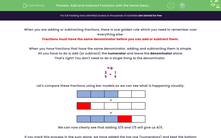# Add and Subtract Fractions with the Same Denominator

In this worksheet, students will add and subtract fractions which share the same denominator, simplifying answers into the simplest forms.Key stage:  KS 4

Year:  GCSE

GCSE Subjects:   Maths

GCSE Boards:   Pearson Edexcel, OCR, Eduqas, AQA,

Curriculum topic:   Number, Fractions, Decimals and Percentages

Curriculum subtopic:   Structure and Calculation Fractions

Difficulty level:#### Worksheet Overview

When you are adding or subtracting fractions, there is one golden rule which you need to remember over everything else:

Fractions must have the same denominator before you can add or subtract them.

When you have fractions that have the same denominator, adding and subtracting them is simple.

All you have to do is add (or subtract) the numerator and leave the denominator alone.

That's right! You don't need to do a single thing to the denominator.

e.g.

 3 5
+
 1 5

Let's compare these fractions using bar models so we can see what is happening visually:We can now clearly see that adding 3/5 and 1/5 will give us 4/5.

If you track this process in the sum alone, we have added the top row (numerators) and kept the bottom number (the denominator) the same, like this:

 3 5
+
 1 = 4 5 5

In this activity, you will practise adding and subtracting fractions with the same denominators, whilst simplifying your answers into their simplest form.

### What is EdPlace?

We're your National Curriculum aligned online education content provider helping each child succeed in English, maths and science from year 1 to GCSE. With an EdPlace account you’ll be able to track and measure progress, helping each child achieve their best. We build confidence and attainment by personalising each child’s learning at a level that suits them.

Get started